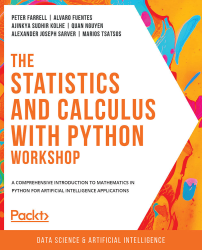•#### The Statistics and Calculus with Python Workshop#### Overview of this book

Are you looking to start developing artificial intelligence applications? Do you need a refresher on key mathematical concepts? Full of engaging practical exercises, The Statistics and Calculus with Python Workshop will show you how to apply your understanding of advanced mathematics in the context of Python. The book begins by giving you a high-level overview of the libraries you'll use while performing statistics with Python. As you progress, you'll perform various mathematical tasks using the Python programming language, such as solving algebraic functions with Python starting with basic functions, and then working through transformations and solving equations. Later chapters in the book will cover statistics and calculus concepts and how to use them to solve problems and gain useful insights. Finally, you'll study differential equations with an emphasis on numerical methods and learn about algorithms that directly calculate values of functions. By the end of this book, you’ll have learned how to apply essential statistics and calculus concepts to develop robust Python applications that solve business challenges.
Preface1. Fundamentals of PythonFree Chapter
2. Python's Main Tools for Statistics3. Python's Statistical Toolbox4. Functions and Algebra with Python5. More Mathematics with Python6. Matrices and Markov Chains with Python7. Doing Basic Statistics with Python8. Foundational Probability Concepts and Their Applications9. Intermediate Statistics with Python10. Foundational Calculus with Python11. More Calculus with Python12. Intermediate Calculus with PythonAppendix# 2. Python's Main Tools for Statistics

Overview

This chapter presents a practical introduction to the main libraries that most statistics practitioners use in Python. It will cover some of the most important and useful concepts, functions, and Application Programming Interfaces (APIs) of each of the key libraries. Almost all of the computational tools that will be needed for the rest of this book will be introduced in this chapter.

By the end of this chapter, you will understand the idea behind array vectorization of the NumPy library and be able to use its sampling functionalities. You'll be able to initialize pandas DataFrames to represent tabular data and manipulate their content. You'll also understand the importance of data visualization in data analysis and be able to utilize Python's two most popular visualization libraries: Matplotlib and Seaborn.